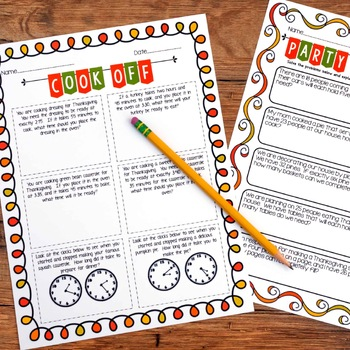# Thanksgiving Math Activities | Print and Digital for Distance Learning3rd - 4th, Homeschool
Subjects
Standards
Resource Type
Formats Included
• PDF
•Google Apps™
Pages
31 pagesThe Teacher-Author indicated this resource includes assets from Google Workspace (e.g. docs, slides, etc.).

### Description

Get no prep and differentiated Thanksgiving themed math lessons with these rigorous and engaging math activities. You will receive multiple versions of six math lessons that require no prep work other than making copies or printing, and they are fun for students!

In this product you'll find:

-Geometric Feast-Students have to set a Thanksgiving table using different shaped platters and serving dishes. This addresses almost all basic polygons mentioned in the Common Core Standards.

-Partitioning Pies-Students will partition six different pies into different numbers of pieces. To give students a little extra enrichment, I've added a Part 2 to the activity, and students will solve fraction word problems using the partitioned pies.

-Cost of Cooking-Students will use an information sheet of nine different food items to solve 12 problems that will require students to use multiple operations to solve one-step and two-step problems. I also included a decimal version for practice adding and subtracting decimals.

-Cook Off-Students will use Thanksgiving recipes to solve six elapsed time problems.

-Party Planning-Students are preparing for their family's Thanksgiving meal, and the preparation leads to five division problems that require students to think about how the remainder effects the problem.

Total Pages
31 pages
Included
Teaching Duration
N/A
Report this Resource to TpT
Reported resources will be reviewed by our team. Report this resource to let us know if this resource violates TpT’s content guidelines.

### Standards

to see state-specific standards (only available in the US).
Partition shapes into parts with equal areas. Express the area of each part as a unit fraction of the whole. For example, partition a shape into 4 parts with equal area, and describe the area of each part as 1/4 of the area of the shape.
Understand that shapes in different categories (e.g., rhombuses, rectangles, and others) may share attributes (e.g., having four sides), and that the shared attributes can define a larger category (e.g., quadrilaterals). Recognize rhombuses, rectangles, and squares as examples of quadrilaterals, and draw examples of quadrilaterals that do not belong to any of these subcategories.
Use multiplication and division within 100 to solve word problems in situations involving equal groups, arrays, and measurement quantities, e.g., by using drawings and equations with a symbol for the unknown number to represent the problem.
Interpret whole-number quotients of whole numbers, e.g., interpret 56 ÷ 8 as the number of objects in each share when 56 objects are partitioned equally into 8 shares, or as a number of shares when 56 objects are partitioned into equal shares of 8 objects each. For example, describe a context in which a number of shares or a number of groups can be expressed as 56 ÷ 8.
Interpret products of whole numbers, e.g., interpret 5 × 7 as the total number of objects in 5 groups of 7 objects each. For example, describe a context in which a total number of objects can be expressed as 5 × 7.